# 一、Vector3.Distance优化

float Vector3.Distance(Vector3 a,Vector b)
{
float sqr_x = (a.x - b.x) * (a.x - b.x);
float sqr_y = (a.y - b.y) * (a.x - b.x);
float sqr_z = (a.z - b.z) * (a.z - b.z);
return Mathf.Sqrt(sqr_x + sqr_y + sqr_z);
}

float SqrDistance(Vector3 a,Vector b)
{
float sqr_x = (a.x - b.x) * (a.x - b.x);
float sqr_y = (a.y - b.y) * (a.x - b.x);
float sqr_z = (a.z - b.z) * (a.z - b.z);
return sqr_x + sqr_y + sqr_z;
}

if(SqrDistance(point1,point2) < minDistance * minDistance)
{
//DoSomething...
}

# 二、除法的优化

float a = 2.333f;
float value = a * 0.25f;//代替a/4

# 三、向量夹角优化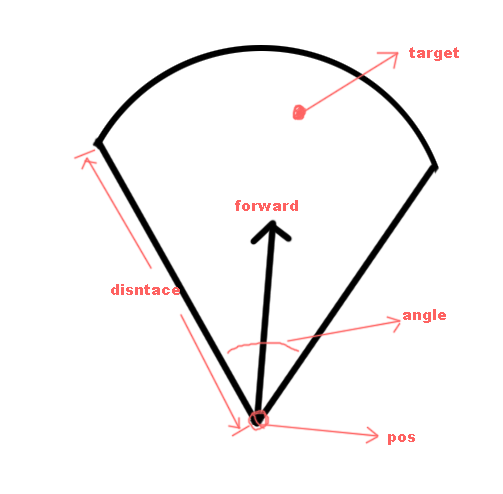//forward是扇形朝向，一般是就是人物朝向（forward本身就是单位向量）
//pos是人物位置
//target是要计算的目标位置
//angle是攻击的角度范围,这是总的角度范围，需要除以2来求出一边的角度。
//distance是扇形的距离
bool CheckFanShape(Vector3 forward,Vector3 pos,Vector3 target,float angle,float distance)
{
Vector3 target_dir = (target - pos).normalize;//算出当前点目标点的向量
float _angle = Vector3.Angle(target_dir, forward);//计算出人物朝向跟目标朝向的角度。用unity的api
Vector3 _disntace = Vector3.Distance(pos,target);//计算两点之间的距离
return _angle <= angle / 2 && _disntace <= diantace;
}

disntace的优化上面提到了。现在要做的就是Angle函数的优化。

float angle = Vector3.Angle (fromVector, toVector); //求出两向量之间的夹角
Vector3 normal = Vector3.Cross (fromVector,toVector);//叉乘求出法线向量
angle *= Mathf.Sign (Vector3.Dot(normal,upVector));  //求法线向量与物体上方向向量点乘，结果为1或-1，修正旋转方向

a·b=x1x2+y1y2=|a||b|cosθ(θ是a，b夹角)

cosθ = a·b/(|a||b|)

向量a * 向量b = x1x2+y1y2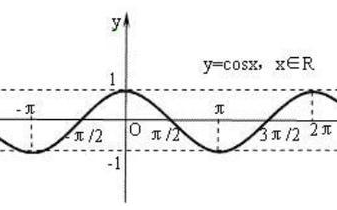∵  |θ1| < |θ2|

∴ cosθ1 > cosθ2  (上面解释的成反比)

∴ 当我们夹角是单位向量时，x1x2+y1y2 > cosθ2

（所有的三角函数，sinθ cosθ  ，因为都是固定的一个数，所以我们可以记好，然后通过读表的方式读取sin和cos的值。一般请教下，θ值都是int就足够了，如果θ 值需要有小数点，那就扩大θ 的精度，表格中保存更多的θ 值。）

Dictionary<int,float> cosTable = new Dictionary<int,float>();
float Utility.Cosf(int angle)
{
return cosTable[angle];
}

Vector3 Utility.Normalize(Vector3 dir)
{
return dir.normalize;//这里留个悬念，后面会讲到这个normalize的优化。
}

bool CheckAngle(Vector3 forward,Vector3 pos,Vector3 target,int angle)
{
//因为这个算的是2D的，所以我们取的是x和z的值
Vector3 dir = Utility.Normalize(target - pos);
float cos_value = forward.x * forward.z + dir.x * dir.z;
float target_cos_value = Utility.Cosf(angle);//注意，这里的angle是除以2以后的。
return cos_value > target_cos_value;
}

# 四、Normalize的优化计算

Vector3 Normalize(Vector3 dir)
{
float sqr_len = dir.x *dir.x + dir.y * dir.y + dir.z * dir.z;
return dir / Mathf.Sqrt(sqr_len);
}

dir * value

//这个函数就是用来计算1/sqr(x)的
float InvSqrt (float x)
{
float xhalf = 0.5f*x;
int i = *(int*)&x;
i = 0x5f3759df - (i>>1);
x = *(float*)&i;
x = x*(1.5f - xhalf*x*x);
return x;
}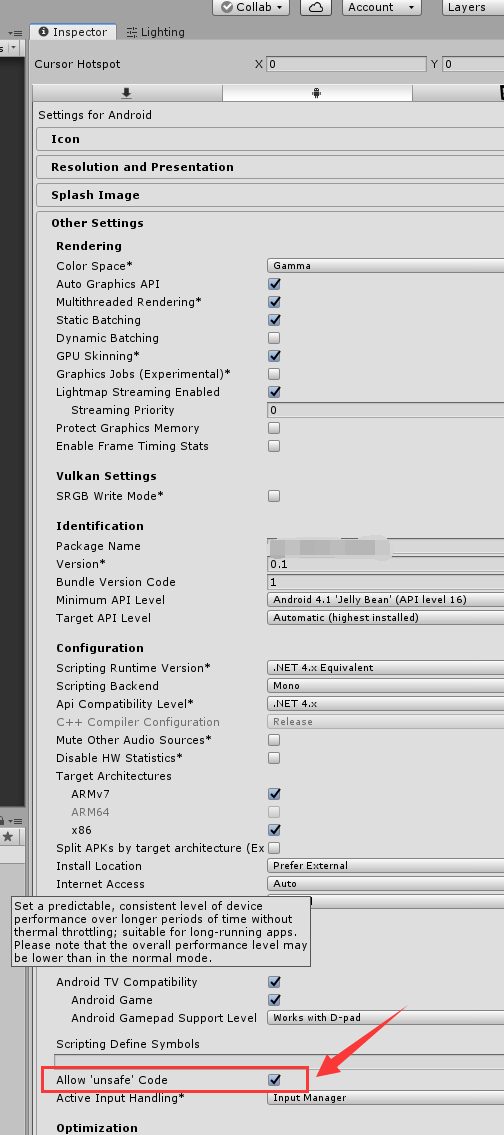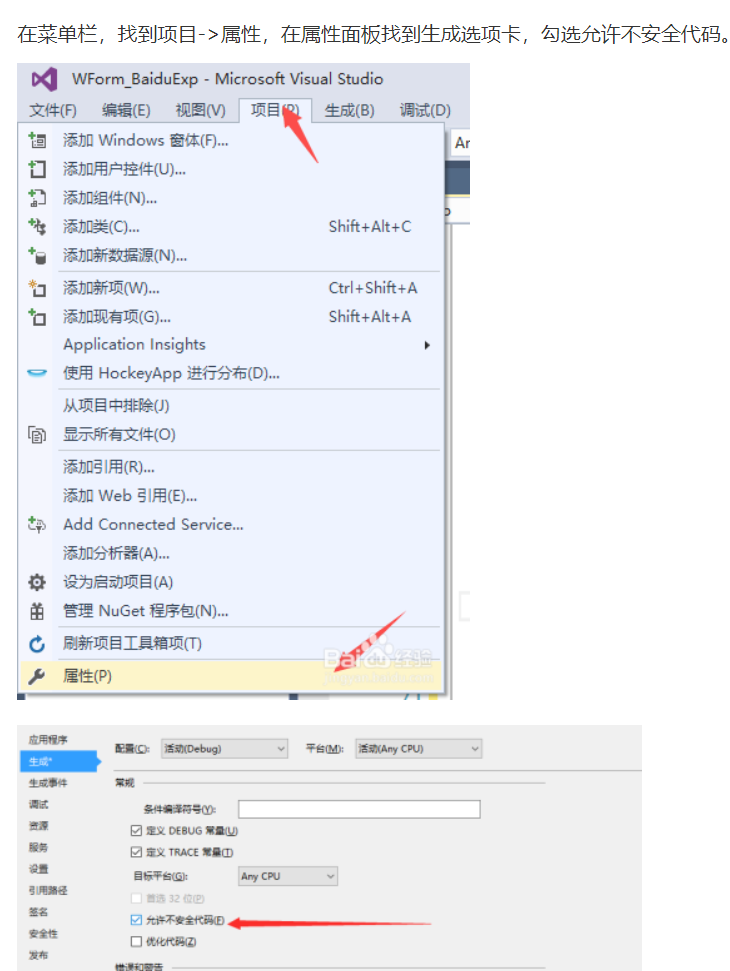# 五、其他一些在项目中可以会经常用到的Vector3公式

①计算多个点的中心点位置。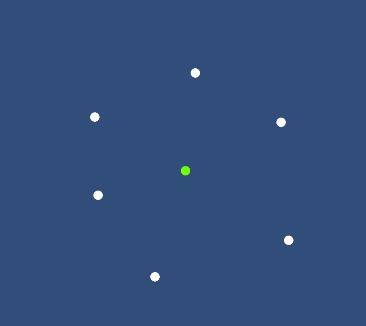Vector3 GetCenter(Vector3[] points)
{
Vector3 center = Vector3.zero;
for (int i = 0; i < points.Length; i++)
{
center += points[i];
}
return center / points.Length;
}

②计算一个点位于一条向量是左边还是右边。（这里是基于2D平面的，所以用Vector2代替，如果是3D的，就没法定义左边右边的含义了。）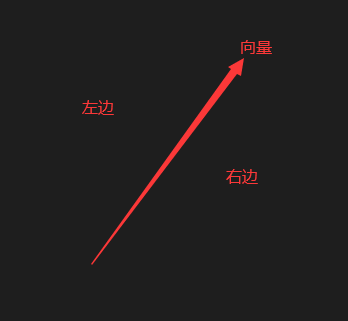enum MyDirectionType//所在方向的偏向哪一边
{
Left,
InLine,
Right
}
//start 是向量的开始位置
//end 是向量的结束位置
//calPoint 是要计算的目标点
MyDirectionType GetDirection(Vector3 start,Vector3 end,Vector3 calPoint)
{
//根据3个点的参数，计算出两条向量
Vector3 dir1 = end - start;
Vector3 dir2 = calPoint - start;
float value = dir1.x * dir2.y - dir1.y * dir2.x;
if (value < 0)
{
return MyDirectionType.Right;
}
else if (value == 0)
{
return MyDirectionType.InLine;
}
else
{
return MyDirectionType.Left;
}
}

06-096万+
10-09663910-0958
05-13916
12-071万+
08-22626
08-052万+
07-07387
04-082405
06-0742